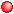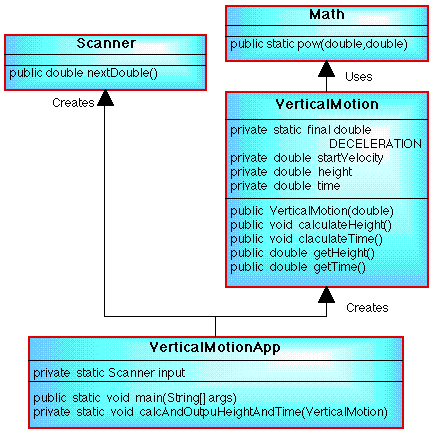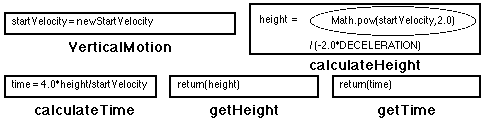# NOTE: This set of www pages is not the set of www pages for the curent version of COMP101. The pages are from a previous version that, at the request of students, I have kept on line.

## CONTENTS

 1. Expressions 2. Boolean expressions 3. Operators 3.1. Precedence of operators 4. Evaluation of expressions 5. Truth tables 6. Lazy evaluation (short circuit evaluation) 7. Statements ` ` 7.1. Assignment statements 7.2. Type equivalence 8.Example problem - vertical motion 8.1. Requirements 8.2. Analysis 8.3. Design 8.4. Implementation 8.5. Testing

The example includes a user defined constant (we have not had an example programme yet which includes user defined constants, although we have used the Math class constant PI). The application class in the example comprises two static methods (main and one other) --- so far all the application classes we have looked at have included only one (main) method. In addition the example, as with the Point_2D class studied earlier, includes the passing of objects as arguments (formal parameters) to methods.

## 1. EXPRESSIONSAn expression in the context of computer programming languages is a formula.Expressions are composed of one or more operands whose values are combined by operators.Typical operators include the maths operators +, -, *, / (there are others).
` `Example: ```numI + (2 * numJ) ```Evaluation of an expression produces a value (an anonymous data item).Expressions can therefore be used to assign values to variables.

## 2. BOOLEAN EXPRESSIONS

Expressions that use Boolean operators (named after the 19th century mathematician George Boole) return a data item of type boolean which can take the values true or false. Well know Boolean operators are given in the Table 1 to the right. The first 6 are sometimes referred to as comparison or relational operators and the remaining 4 as logical operators. Examples of Boolean expressions:

```operand1 < 50

operand2 == 0

operand3 > = 10 & operand3 < = 20

operand3 < 10 | operand3 > 20

operand1 < 20.0 & (operand2 != 'q' |
myInstance.testFun(operand1,
operand4) == 1)
```
OperationJava encoding
Equals ==
Not equals !=
Less than <
Greater than >
Less than or equal to <=
Greater than or equal to >=
Logical and &, &&
Logical or |, ||
Exclusive Logical or ^
Logical not !

Table 1. Boolean operations

These expressions can be interpreted as follows:

1. Return true if value for data item operand1 is less than 50, otherwise return false.
` `
1. Return true if value for data item operand2 is equal to 0, otherwise return false.
2. Return true if value for data item operand3 is greater than or equal to 10 and less than or equal to 20 (i.e. operand3 is within the range 10..20), otherwise return false.
3. Return true if value for data item operand3 is less than 10 or greater than 20 (i.e. operand3 not within the range 10..20), otherwise return false.
4. Return true if value for data item operand1 is less than 20.0 and either the value for operand2 is not the character 'q' or the value returned by the test function (which has two arguments operand1 and operand4) is equal to 1, otherwise return false.

The comparison operators may be applied to both numeric types and characters. The result of comparison on characters will depend on the unicodes associated with the characters in question. For example 'B' < 'a' is true ('B' has a unicode of 66 while 'a' has a unicode of 97).Figure 2: George Boole (1)

## 3. OPERATORSThe number of operands that an operator requires is referred to as its arity.Operators with one operand are called monadic or unary.Operators with two operands are called dyadic or binary.Some programming languages (but not Java) support ternary operators.Operators can also be classified as prefix, infix or postfix.

### 3.1. Precedence of operatorsOperators are ordered according to which should be applied first. We say that operators have precedence.We also consider levels of precedence to exist.Low-level operators have precedence (are evaluated first) over high-level operators.Consequently we say that low-level operators bind their operands more strongly than high-level operators.Operators on the same level have the same precedence.

Table 2 shows the precedence levels/ordering of a number of common operators starting with low level operators and progressing down through higher level operators.

The order of execution of operators on the same level is controlled by associativity:Left-associative operators are executed from left to right.Right-associative operators are executed from right to left.

If an operator is non-associative it cannot be used in a sequence. For example the comparison operator (==) is non-associative. Thus we cannot write a boolean expression of the form:

```sideA == sideB == sideC
```
` `
LevelOperator
0( ) (brackets)
1+, - (unary plus and minus), ++, --, casts
.
` `
3*, /, %
4+ (concatenation), +, -
.Comparison operators
.! (Logical not)
.&, && (Logical and)
.|, ||, ^ (Other logical operators)
.= (assignment)

Table 2. Precedence ordering of operations

```((sideA == sideB) & (sideB == sideC))
```

Note that the & operator is associative (as are the &&, |, || and ^ operators).

Java also provides at least one triadic operator (an operator that has three operands):

```operand1 ? opeerand2 : operand3

but what this does is beyond the scope of this lecture.
```

## 4. EVALUATION OF EXPRESSION

 Consider the Java code presented in Table 3. (Ignoring the fact that we should not be using single letter identifiers) we can evaluate the expression a+b*c-d/e according to the Java precedence and association rules for the individual operators. Replacing the variables with their values we get: ```1+2*3-4/5 ``` The expression contains two level 3 operators (* and /), and two level 4 operators (+ and -). Level 3 operators are evaluated before level 4 operators, and all Java arithmetic operators are left associative. Thus the first sub expression evaluated is b*c, i.e. 2*3 because this is the first operator (reading from left to right) with the lowest precedence. This will give 6. We now have: ```1+6-4/5 ``` ` ` Next we evaluate 4/5 (d/e) which will give 0 (remember we are doing integer division). The expression now becomes: ```1+6-0 ``` We now evaluate the 1+6 part to give 7 and: ```7-0 ``` will give 7. When working with expressions of the form shown in Table 3 it is a good idea to include parenthesis so as to make the expression as clear as possible. Thus: ```a + (b*c) - (d/e) ``` Note also that the division operator ('/') when used with operands of type int produces "integer division"; this is why in the above example 4/5 gives the result 0.
 ``` // OPERATOR EVALUATION // Frans Coenen // Monday 12 March 1999 // The University of Liverpool, UK class OperatorEvaluation{ // --------------- METHODS ------------------ /* MAIN METHOD: */ public static void main(String[] args) { int a = 1; int b = 2; int c = 3; int d = 4; int e = 5; int answer; answer = a+b*c-d/e; System.out.println("Answer = " + answer); } } ```

Table 3: Operator evaluation program

## 5. TRUTH TABLES

 The operation of the logical operators can be illustrated using what are known as truth tables. Four such tables are given in Figure 1, one for each of the logical operators given above. If we consider the table for the logical "and", this is an infix binary operator and this has two operands (as do the logical "or" and "exclusive or", but not the "not" which is prefix unary operator). The inputs can be true or false (if the inputs are literals then they are automatically true). Thus for the binary operators we have four possible combinations of input which are given in the left two columns of the Tables in Figure 1. ` ` For a logical "and" to return true, both inputs must be true --- as evidenced by the table. The distinction between a logical "or" and an "exclusive or" is that in the first case one or other or both operands must be true, while in the second case one or other but not both operands must be true. The above has implications for testing. Given a logical "and", "or" or "exclusive or" we should derive test cases for all four possible combinations. Similarly, given a "logical not" we should test for both combinations.
operand1operand2operand1 & operand2
true true true
true falsefalse
falsetrue false
falsefalsefalse
Logical and
operand1operand2operand1 | operand2
true true true
true falsetrue
falsetrue true
falsefalsefalse
Logical or
operand1operand2operand1 ^ operand2
true true false
true falsetrue
falsetrue true
falsefalsefalse
Logical exclusive or
operand1!operand1
true false
falsetrue
Logical not

Figure 1: Truth tables

## 6. LAZY EVALUATION (SHORT CIRCUIT EVALUATION)

 Consider the expression: ```operand1 & operand2 ``` If operand1 is false then the expression will return false regardless of the value of operand2. Similarly in the case of: ```operand1 | operand2 ``` If operand1 is true then the expression will return true regardless of the value of operand2. ` ` In both cases the second operand has been evaluated needlessly. Java therefore provides "short cut" versions of & and |, namely && and || which avoid this needless evaluation. Thus: ```operand1 && operand2 operand1 || operand2 ``` The mechanism where by we only evaluate operands if we need to is called lazy evaluation or short circuit evaluation. Some example code illustarting the distinction between the & and && is available.

## 7. STATEMENTS

Statements are the commands in a language that perform actions and change the "state" (the term is used for historical reasons). The state of a program is described by the values held by its variables at a specific point during its execution. We can identify six broad categories of statement common to most OOP languages:Assignment --- change the value (state) of a field.Method calls --- cause a method to be "performed", in object oriented parlance (although not in Java) this is sometimes referred to as message passing.I/O (Input/Output) --- statements to control input and output (from various sources and to various destinations).
` `Selection --- choose between alternative statements or groups of statements to be "performed".Repetition --- "perform" a certain statement or group of statements in an iterative manner until some end condition is met.Exception --- detect and react to some unusual circumstance.

A Similar categorisation of statements is found in imperative programming languages. Statements are usually separated by some operator (symbol), in Java this is the semi-colon character (;).

### 7.1 Assignment statements

We have met the concept of assignment previously. The value of a variable can always be modified using an assignment statement:

```NUMBER := 2;
```
` `The operator used here is referred to as an assignment operator.The left hand operand is called the destination and the right hand operand the source.The source of a value in an assignment is usually an expression referred to as an assignment expression.

### 7.2. Type equivalenceExpressions require their operands to be of a certain type.The compilers will check for this. This is called equivalence testing.
` `

Where necessary, in Java, we can sometimes "force" type equivalence using a type conversions (cast).

```T(N)
```

## 8. EXAMPLE PROBLEM --- VERTICAL MOTION

where u = start velocity and g = deceleration due to gravity, to calculate the maximum height (h), and:

```t = (4 x h) / u
```

### 8.2 Analysis

A class diagram for the proposed solution is given in Figure 3.

### 8.3 Design

From Figure 3 the design requires two classes, Verticalmotion and VertMotionApp.

#### 8.3.1 VerticalMotion ClassFigure 3: Vertical motion class diagram

 Field Summary private static final double DECELERATION            A class constant with the value -10.0. private double startVelocity            An instance variable describing the start velocity (u) of a vertically thrown ball. private double height            An instance variable in which to store the maximum height reached (h) of a vertically thrown ball. private double time            An instance variable in which to store the duration (t) of a vertically thrown ball from initial launch to returning to the launch point.

 Constructor Summary VerticalMotion(double newStartVelocity)            Constructor to create an instance of the class VerticalMotion given a start velocity.

 Method Summary public void calculateHeight()            Method to determine maximum height of ball using given identity. The items given in the data table to the right will be used. public void calculateTime()            Method to calculate the time taken from "launching" the ball from the ground till it returns to the ground. Calculation is done using the given identity. public double getHeight()            Method to return height (a private data member). public double getTime()            Method similar to getHeight method (above) to return time (a private data member).

Nassi-Shneiderman charts for the above methods are presented in Figure 4.Figure 4: Nassi-Shneiderman charts for VerticalMotion class methods

#### 8.3.2 VertMotionApp Class

 Field Summary private static Scanner input            A class instance to facilitate input from the input stream.

 Method Summary public static void main(String[] args)            Main method to allow user to input a start velocity, create an instance of the type VerticalMotion and then call the calcAndOutputHeightAndTime method described below. private static void calcAndOutputHeightAndTime(VerticalMotion ball)            Class method to determine the height and time to "return to the ground" of the particular ball passed to it as a formal parameter.

Note that alculations are made through calls to the calculateHeight and calculateTime methods contained in the VerticalMotion class. Once calculated the results of the calculation are output. Both the height and time data items are private instance variables belonging to the VerticalMotion class so we need to make use of the getHeight and getTime methods (also defined in the VerticalMotion class) to obtain these values.

Nassi-Shneiderman charts for the above methods are presented in Figure 5.Figure 5: Nassi-Shneiderman charts for VertMotionApp class methods

### 8.4. Implementation

#### 8.4.2 VerticalMotion class

 ```// VERTICAL MOTION // Frans Coenen // Monday 16 March 1999 // The University of Liverpool, UK class VerticalMotion{ // --------------- CONSTANTS ------------------ private static final double DECELERATION = -10.0; // ----------------- FIELDS ------------------- private double startVelocity; private double height; private double time; // --------------- CONSTRUCTORS --------------- /* Constructor */ public VerticalMotion(double newStartVelocity) { startVelocity = newStartVelocity; } // ----------------- METHODS ------------------ /* Calculate height */ public void calculateHeight() { height = Math.pow(startVelocity,2.0)/(-2.0*DECELERATION); } /* Calculate time */ public void calculateTime() { time = 4*height/startVelocity; } /* Get height */ public double getHeight() { return(height); } /* Get time */ public double getTime() { return(time); } } ```

Table 4: Vertical motion class hierarchy implementation

Note the precedences that apply in the calculateHeight method:

```Math.pow(startVelocity,2.0)/
(-2.0*DECELERATION)
```

We would first evaluate the * because this is encased in parenthesises which have precedence of 0 --- -2.0*-10.0 = 20. Then we would evaluate the / operator; if we assume a start velocity of 10, then 100/20 = 5 (the height in this case). However if we omitted the parenthesises:

```Math.pow(startVelocity,2.0)/
-2.0*DECELERATION
```

the division would be evaluated first (100/-2 = -50), and then the multiplication (-50*-10 = 500) --- not the desired result at all!

#### 8.4.3 VertMotionApp class

 ```// VERTICAL MOTION APPLICATION // Frans Coenen // Monday 16 March 1999 // Revised: Monday 4 July 2005 // The University of Liverpool, UK import java.util.*; class VertMotionApp{ // ------------------- FIELDS ------------------------ private static Scanner input = new Scanner(System.in); // ----------------- METHODS ------------------ /* Main method */ public static void main(String[] args) { // Input start velocity System.out.println("Enter start velocity:"); double startVel = input.nextDouble(); // Create Vertical Motion Instance VerticalMotion vertMotionOfBall = new VerticalMotion(startVel); // Calculate and output height and time calcAndOutputHeightAndTime(vertMotionOfBall); } /* Calculate and output height and time */ private static void calcAndOutputHeightAndTime(VerticalMotion ball) { // Make calculations ball.calculateHeight(); ball.calculateTime(); // Output results System.out.println("Height = " + ball.getHeight()); System.out.println("Time = " + ball.getTime()); } } ```

Table 5: Vertical motion application

Note: the method calcAndOutputHeightAndTime is defined as a static method here so that it is class method, if we did not do this (i.e. we defined it as a instance method) we would first have to create an instance of the class VertMotionApp (using the default constructor that is automatically created by Java). There is nothing that prevents us from doing this, it is simply more convenient in this case to use a class method.

### 8.5. Testing

Use Arithmetic testing to test negative, zero and positive input values. An appropriate set of test cases is given in the table (right). The Error is included because we expect a divide by zero error --- computers cannot resolve expressions involving a division by the number 0 and consequently a divide by zero error results.

TEST CASEEXPECTED RESULT
startVelocitymaxHeighttotalTime
50.0125.0 10.0
0.0 0.0Error
-50.0125.0-10.0

Finally include some data validation test cases.

## REFERENCES

Created and maintained by Frans Coenen. Last updated 10 February 2015